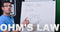Ohm’s law is used to describe the relationship between Voltage (V), Current (I) and Resistance (R). In this video Kevin explains how the relationship works and shows you a practical demonstration. There is a further explanation and some questions to test your understanding below.
If you know any of two of these values it is possible to calculate the third. Ohm’s law can be arranged in the following three ways depending on what you want to calculate. V = I x R I = V / R R = V / I Note: V = Voltage in Volts, I = Current in Amps, R = Resistance in Ohm’s

## The Ohm’s Law Triangle

A very useful tool that can be used when doing one of the above calculations is the Ohm’s law triangle shown below. The triangle is used by covering the item which you wish to calculate. The two items that are not covered will then be left in the arrangement required to calculate the covered item. For example if you want to calculate R you would cover that item. The two remaining items are arranged as V/I. This is the formula to calculate R.## Questions

Voltage: Using the following Currents and Resistances calculate the corresponding Voltage.
 Question 1: I = 5 Amps R = 1 Ohm Answer = Question 2: I = 3 Amps R = 4 Ohm Answer = Question 3: I = 2 Amps R = 3 Ohm Answer =
Current: Using the following Voltages and Resistances calculate the corresponding Current.
 Question 4: V = 5 Volts R = 1 Ohm Answer = Question 5 V = 12 Volts R = 4 Ohm Answer = Question 6: V = 24 Volts R = 12 Ohm Answer =
Resistance: Using the following Voltages and Currents calculate the corresponding Resistances.
 Question 7: V = 5 Volts I = 5 Amps Answer = Question 8: V = 3 Volts I = 6 Amps Answer = Question 9: V = 50 Volts I = 10 Amps Answer =

Download a pdf version of this page hereLearn more about the author